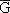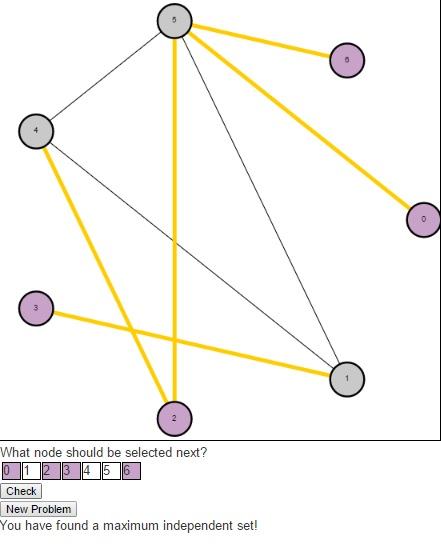# Independent Set Puzzles

In this post, I want to return to the idea of NP-Complete problems. There is a more technical, more formal definition that I can refer you to, but I like to refer to the images from Garey and Johnson’s “Computers and Intractability: A Guide to the Theory of NP Completeness”. The images helps to understand the difficulty of NP-Complete problems by presenting two images. The first image shows a single individual speaking to someone saying that he has been unable to solve the problem. The second image shows that same individual speaking to the same person behind the desk, but saying that not only was he unable to solve the problem but neither was a long line of people. The theory of NP Complete problems revolves around the concept that if an efficient algorithm exists for an NP-Complete problem, then an efficient algorithm exists for all problems in the class NP.

Today, I would like to present a puzzle I created to play with the Independent Set problem. This is the problem where we are given a graph G = (V, E) and are asked to find a maximum set of vertices S such that there is no edge in the graph G between any two vertices in S. The decision version (the problem asking whether there is an independent set of size k) of this problem is NP-Complete, so the known algorithms for problem either have a slow running time, or do not solve it exactly.

This problem is very related to another puzzle I posted last year called the clique problem. In fact, Karp originally proved that Clique was NP-Complete by showing that if Clique could be solved efficiently, then Independent Set could be solved efficiently. He did this by constructing a second graph [G bar], called the compliment of G (containing the same vertices in G, along with the edges that are not present in G. Any edge present in G will not be present in). Then he showed that the nodes representing a maximum clique in G would represent a maximum independent set in. He had already shown that Independent Set was NP-Complete, which meant that both Independent Set and Clique were among the most difficult problems within the class known as NP.The puzzle begins with an undirected graph and asks users to find a maximum independent set. Users should click on the numbers in the table below the graph indicating the nodes they wish to select in their independent set (purple indicates that the node is selected, gray indicates that it is not). Once a user have a potential solution, they can press the “Check” button to see if their solution is optimal. If a user is having trouble and simply wishes to see the maximum independent set, they can press the “Solve” button. And to generate a new problem, users can press the “New Problem” button.As a result of this relationship between the Clique problem and the Independent Set problem, the Bron-Kerbosch algorithm that was used to find maximum cliques previously can also be used here.

## One thought on “Independent Set Puzzles”

This site uses Akismet to reduce spam. Learn how your comment data is processed.